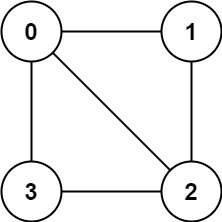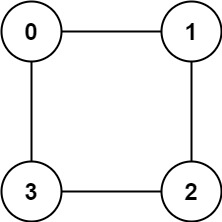# 判断二分图

## 785. 判断二分图 (Medium)

• 不存在自环（`graph[u]` 不包含 `u`）。
• 不存在平行边（`graph[u]` 不包含重复值）。
• 如果 `v``graph[u]` 内，那么 `u` 也应该在 `graph[v]` 内（该图是无向图）
• 这个图可能不是连通图，也就是说两个节点 `u``v` 之间可能不存在一条连通彼此的路径。```输入：graph = [[1,2,3],[0,2],[0,1,3],[0,2]]```输入：graph = [[1,3],[0,2],[1,3],[0,2]]

• `graph.length == n`
• `1 <= n <= 100`
• `0 <= graph[u].length < n`
• `0 <= graph[u][i] <= n - 1`
• `graph[u]` 不会包含 `u`
• `graph[u]` 的所有值 互不相同
• 如果 `graph[u]` 包含 `v`，那么 `graph[v]` 也会包含 `u`

### 相关话题

[深度优先搜索] [广度优先搜索] [并查集] []